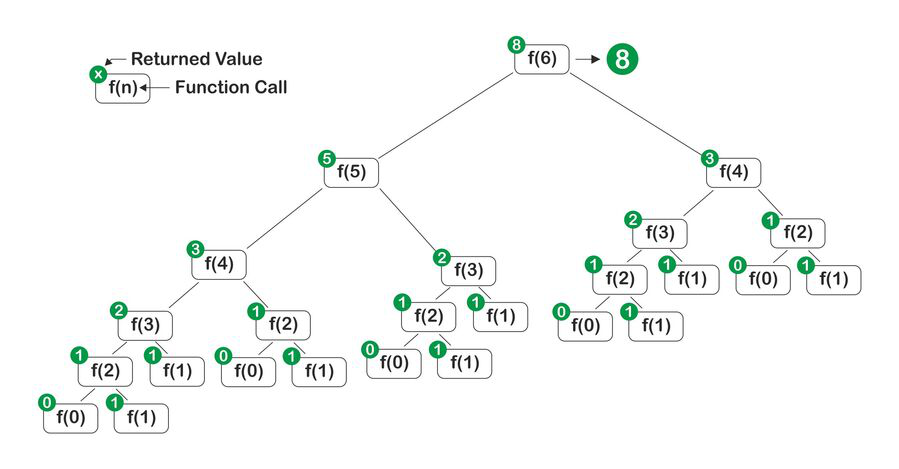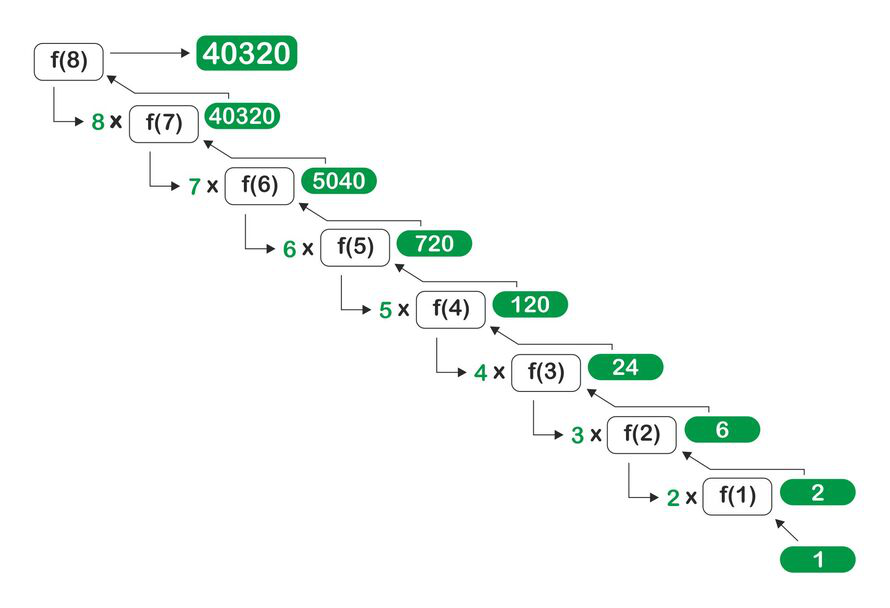Related Articles

# Dart – Recursion

• Last Updated : 12 Mar, 2021

Recursion in any programming language means a function making a call to itself. It is used to solve large complex problems by breaking them into smaller subproblems. Dart also implements recursion similarly.

In a recursive function, the function calls itself repeatedly until a base condition is reached. The function basically has two parts.

• Recursive: The recursive part is called again and again with a smaller subproblem.
• Base: The base condition is checked every time a function call is made. If the function is in a base condition it is used to provide a solution.

Recursion uses a stack to store the values/ result of the subproblems to be later returned to the main problem.

For an in-depth review of recursion visit: https://www.geeksforgeeks.org/recursion/

Syntax:

```void gfgRec()
{
// Base code....

gfgRec();

// Some code...
}

void main()
{
gfgRec();
}```

Example:

We will be using a program for the nth Fibonacci number to demonstrate recursion in dart –

## Dart

 `int` `Fib(``int` `n){``  ``if``(n<=1) ``//Base Condition``    ``return` `n;``  ``return` `Fib(n-1)+Fib(n-2);``}`` ` ` ` `void` `main() {``  ``print(Fib(6));``}`

Output:

`8`

Explanation:From the figure, we can see that the call to the function with n = 6 as a parameter, in turn, calls itself with smaller values 5 and 4. They themselves call the function with the smaller values until it reaches the base condition. When the base condition is met the function start returning the values. So the final result comes out to be 8 which is the sum of the call to 5 and 4.

In the case of Recursion, the problem needs to get smaller with every new function call as the problem has to get terminated to return a result. This condition is called as Termination condition in Recursion. In case the problem does not terminate it can lead to an infinite loop and as we can see that these function call uses stack memory to store the temporary values they may use a lot of memory. If the base condition is not defined or is unable to reach then the function can cause a stack overflow.

• The problem solution using recursion is very concise and short.
• They are readily used with problems related to trees and graphs.
• Reduces the time complexity of problems.
• Reduces unnecessary function calling.

• The problem uses a lot of space.
• Logic can be complex to understand.
• When stuck it is hard to debug.

Now we will be using another example to get a better understanding of its implementation in dart.

Example:

We will be writing a program to find the factorial of a number using recursion.

## Dart

 `int` `Fact(``int` `n){``  ``if``(n<=1) ``//Base Condition``    ``return` `1;``  ``return` `n*Fact(n-1);``}`` ` ` ` `void` `main() {``  ``print(Fact(8));``}`

Output:

`40320`

Explanation:From the figure, we can infer that the Fact function calls itself until the base condition is reached i.e. we returned the function with a multiplication of value ‘n’ with the value of a function with value ‘n-1’ until the value of n becomes equals to 1. At last, we return the value of the desired function.

My Personal Notes arrow_drop_up